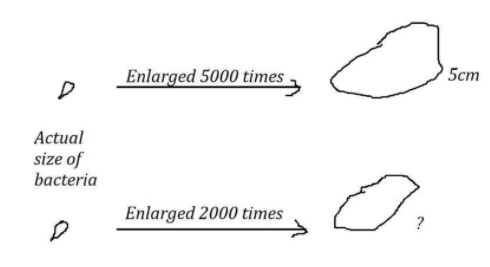QuestionAnswers

# A photograph of a bacteria enlarged $50,000$ times attains a length of $5cm$. Now, find out the actual length of the bacteria and if the photograph is enlarged $20,000$ times only, what would be its enlarged length?

Hint:The length of the photograph is given for a certain value of enlargement. But to get the actual value of the length of the bacteria, we need to divide with that certain value. And after that to find out for any kind of other enlargement value, directly you can multiply that with the actual length of the bacteria.

When we clearly look at the question, they mentioned that the bacteria when it is enlarged for $50,000$ times it attains the length of $5cm$.
So, if we want to find out the actual length of the bacteria then we should divide the length obtained value with the how many times the bacteria are enlarged.In simple words, it gives the length of the bacteria when the photograph is enlarged for unity it means no enlargement at all.
So, from the data we can obtain that the length of bacteria after enlargement is $5cm$ and enlarged $50,000$ times. Substituting these values, we get as follows:
Actual length of the bacteria $= \dfrac{5}{{50000}}$
$= \dfrac{1}{{10000}} \\ = {10^{ - 4}}cm \\$
As per now, we got the actual length of the bacteria and that is ${10^{ - 4}}cm$.
Now we can able to calculate the length of bacteria for any amount of enlargement value by simply multiplying with the unity value that we obtained recently.
In the second part of the question, we are asked to find out the length of the bacteria if the photograph is enlarged for the $20,000$ times.
So, just multiply this enlargement value with the actual length of the bacteria.
We get as follows:
When the photograph of a bacteria is enlarged $20,000$ times attains a length $= 20000 \times {10^{ - 4}}= 2cm$

So, the bacterial will be visible with $2cm$ length when it is enlarged for the $20,000$ times.

Note:You are able to solve this problem in other ways also. We need to look at the proportions between the times that enlarged for data is given and the time enlarged for data asked. In this problem firstly it is enlarged by $50,000$ times but asked for $20,000$ times. The proportional ratio is $\dfrac{2}{5}$. So, simply multiplying $5cm$ with $\dfrac{2}{5}$, we will get the same answer.